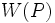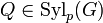# Equivalence of normality and characteristicity conditions for conjugacy functor

This article gives a proof/explanation of the equivalence of multiple definitions for the term conjugacy functor that gives a normal subgroup
View a complete list of pages giving proofs of equivalence of definitions
This article gives a proof/explanation of the equivalence of multiple definitions for the term characteristic p-functor that gives a characteristic subgroup
View a complete list of pages giving proofs of equivalence of definitions

## Statement

### For conjugacy functors arising from characteristic p-functors

Suppose$p$ is a prime number and$G$ is a finite group such that$W$ is a conjugacy functor for$G$ for the prime$p$ arising from a characteristic p-functor. The following are equivalent:

1. For every pair of$p$-Sylow subgroups$P,Q$ of$G$,$W(P) = W(Q)$.
2. For every pair of$p$-Sylow subgroups$P,Q$ of$G$,$W(P)$ is a normal subgroup of$Q$.
3. Each of these:
•$W$ is a weakly closed conjugacy functor and there exists a$p$-Sylow subgroup$P$ of$G$ such that$W(P) \le O_p(G)$ where$O_p(G)$ is the p-core of$G$.
•$W$ is a weakly closed conjugacy functor and for every$p$-Sylow subgroup$P$ of$G$,$W(P) \le O_p(G)$ where$O_p(G)$ is the$p$-core of$G$.
4. Each of these:
• There exists a$p$-Sylow subgroup$P$ of$G$ such that$W(P)$ is a characteristic subgroup of$G$.
• For every$p$-Sylow subgroup$P$ of$G$,$W(P)$ is a characteristic subgroup of$G$.
5. Each of these:
• There exists a$p$-Sylow subgroup$P$ of$G$ such that$W(P)$ is a normal subgroup of$G$.
• For every$p$-Sylow subgroup$P$ of$G$,$W(P)$ is a normal subgroup of$G$.

### For conjugacy functors not arising from characteristic p-functors

All parts of the statement above are equivalent with the exception of (4).

## Proof

### Characteristic p-functor case

The proofs of the equivalence of both versions of (3) with each other, both versions of (4) with each other, and both versions of (5) with each other follow from Fact (1).

The equivalence of (1), (2), and (3) essentially follows from Fact (2).

(1) implies (4) is easy to see if it is a characteristic p-functor: we have given a definition: "pick a Sylow subgroup and apply$W$ to it" that always yields the same subgroup. This is a subgroup-defining function and hence gives a characteristic subgroup of$G$.

(4) implies (5) follows from Fact (3).

Finally, we show (5) implies (2): then$W(P) \le O_p(G)$ on account of being a normal$p$-subgroup. Hence,$W(P) \le Q$ for all$Q \in \operatorname{Syl}_p(G)$, and thus by Fact (4), it is normal in$Q$.

### General case

In the general case, the proof proceeds the same way, but instead of showing (1) implies (4) and (4) implies (5), we directly show that (1) implies (5).